# Young tableau

of order $m$

A Young diagram of order $m$ in whose cells the different numbers $1,\ldots,m$ have been inserted in some order, e.g. $$\fbox{5,7,9,4|8,2,1|3|6}$$

A Young tableau is called standard if in each row and in each column the numbers occur in increasing order. The number of all Young tableau for a given Young diagram $t$ of order $m$ is equal to $m!$ and the number of standard Young tableaux is $$\frac{m!}{\prod\lambda_{ij}}$$

where the product extends over all the cells $c_{ij}$ of $t$ and $\lambda_{ij}$ denotes the length of the corresponding hook.

In Western literature the phrase Ferrers diagram is also used for a Young diagram. In the Russian literature the phrase "Young tableau" ( "Yunga tablitsa" ) and "Young diagram" ( "Yunga diagramma" ) are used precisely in the opposite way, with "tablitsa" referring to the pictorial representation of a partition and "diagramma" being a filled-in "tablitsa" .

Letdenote a partition of(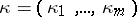,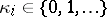,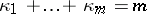) as well as its corresponding Young diagram, its pictorial representation. Letbe a second partition of. A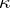-tableau of typeis a Young diagramwith its boxes filled with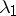's,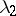's, etc. For a semi-standard-tableau of typethe labelling of the boxes is such that the rows are non-decreasing (from left to right) and the columns are strictly increasing (from top to bottom). E.g.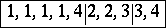is a semi-standard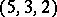-tableau of type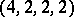. The numbers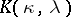of semi-standard-tableaux of typeare called Kostka numbers.

To each partitionofthere are associated two "natural" representations of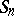, the symmetric group onletters: the induced representation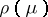and the Specht module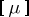. The representationis: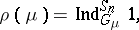whereis the trivial representation of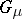andis the Young subgroup ofdetermined by,, where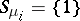if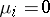and otherwise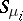is the subgroup of permutations on the letters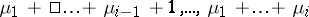.

The groupacts on the set of all-tableaux by permuting the labels. Two-tableaux are equivalent if they differ by a permutation of their labels keeping the sets of indices in each row set-wise invariant. An equivalence class of-tableaux is a-tabloid. The action ofon-tableaux induces an action on-tabloids, and extending this linearly over a base fieldgives a representation ofwhich is evidently isomorphic to. The dimension ofis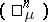. Given a-tableau, letbe the following element of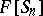: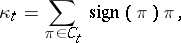where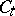is the column-stabilizer of, i.e. the subgroup ofof all permutations that leave the labels of the columns ofset-wise invariant.

The Specht module,, ofis the submodule ofspanned by all the elements, where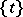is the tabloid ofandis a-tableau. Over a field of characteristic zero the Specht modules give precisely all the different absolutely-irreducible representations of. By Young's rule, the number of times that the Specht moduleoveroccurs (as a composition factor) inis equal to the Kostka number. If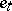is the Young symmetrizer of a-tableau, then the Specht module defined by the underlying diagram is isomorphic to the ideal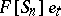of. This is also (up to isomorphism) the representation denoted by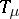in Representation of the symmetric groups. Cf. Majorization ordering for a number of other results involving partitions, Young diagrams and tableaux, and representations of the symmetric groups.

How to Cite This Entry:
Young tableau. Encyclopedia of Mathematics. URL: http://encyclopediaofmath.org/index.php?title=Young_tableau&oldid=39847
This article was adapted from an original article by E.B. Vinberg (originator), which appeared in Encyclopedia of Mathematics - ISBN 1402006098. See original article